# Volume of a Solid made of Cubes with Unit Fraction Edge Lengths Online Quiz

Following quiz provides Multiple Choice Questions (MCQs) related to Volume of a Solid made of Cubes with Unit Fraction Edge Lengths. You will have to read all the given answers and click over the correct answer. If you are not sure about the answer then you can check the answer using Show Answer button. You can use Next Quiz button to check new set of questions in the quiz.Q 1 - Find the volume of following solid of cubes with unit fraction edge lengths. Each prisms unit is measured in cm (not to scale)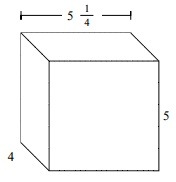### Explanation

Step 1:

Solid of cubes with unit fraction edge lengths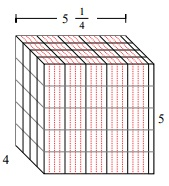Step 2:

Volume V = l w h = $5 \frac{1}{4}$ × 4 × 5

= 105 cu cm

Q 2 - Find the volume of following solid of cubes with unit fraction edge lengths. Each prisms unit is measured in cm (not to scale)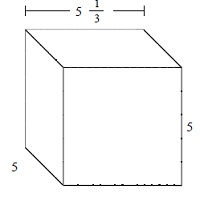### Explanation

Step 1:

Solid of cubes with unit fraction edge lengths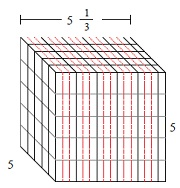Step 2:

Volume V = l w h = $5 \frac{1}{3}$ × 5 × 5

= $133 \frac{1}{3}$ cu cm

Q 3 - Find the volume of following solid of cubes with unit fraction edge lengths. Each prisms unit is measured in cm (not to scale)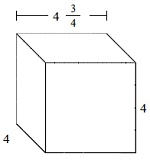### Explanation

Step 1:

Solid of cubes with unit fraction edge lengths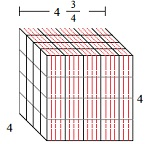Step 2:

Volume V = l w h = 4 $\frac{3}{4}$ × 4 × 4

= 76 cu cm

Q 4 - Find the volume of following solid of cubes with unit fraction edge lengths. Each prisms unit is measured in cm (not to scale)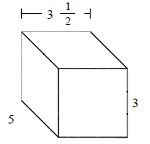### Explanation

Step 1:

Solid of cubes with unit fraction edge lengthsStep 2:

Volume V = l w h = $3 \frac{1}{2}$ × 5 × 3

= $52 \frac{1}{2}$ cu cm

Q 5 - Find the volume of following solid of cubes with unit fraction edge lengths. Each prisms unit is measured in cm (not to scale)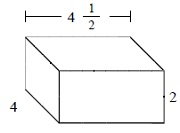### Explanation

Step 1:

Solid of cubes with unit fraction edge lengths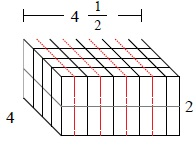Step 2:

Volume V = l w h = $4 \frac{1}{2}$ × 4 × 2

= 36 cu cm

Q 6 - Find the volume of following solid of cubes with unit fraction edge lengths. Each prisms unit is measured in cm (not to scale)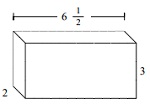### Explanation

Step 1:

Solid of cubes with unit fraction edge lengths Volume = 39 cu cm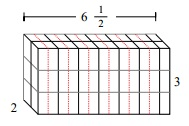Step 2:

Volume V = l w h = $6 \frac{1}{2}$ × 2 × 3

= 39 cu cm

Q 7 - Find the volume of following solid of cubes with unit fraction edge lengths. Each prisms unit is measured in cm (not to scale)### Explanation

Step 1:

Solid of cubes with unit fraction edge lengthsStep 2:

Volume V = l w h = $3 \frac{2}{4}$ × 4 × 3

= 42 cu cm

Q 8 - Find the volume of following solid of cubes with unit fraction edge lengths. Each prisms unit is measured in cm (not to scale)### Explanation

Step 1:

Solid of cubes with unit fraction edge lengths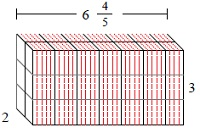Step 2:

Volume V = l w h = $6 \frac{4}{5}$ × 2 × 3

= $40 \frac{4}{5}$ cu cm

Q 9 - Find the volume of following solid of cubes with unit fraction edge lengths. Each prisms unit is measured in cm (not to scale)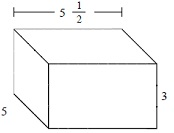### Explanation

Step 1:

Solid of cubes with unit fraction edge lengths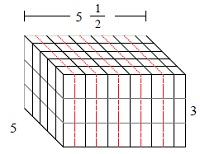Step 2:

Volume V = l w h = $5 \frac{1}{2}$ × 5 × 3

= $82 \frac{1}{2}$ cu cm

Q 10 - Find the volume of following solid of cubes with unit fraction edge lengths. Each prisms unit is measured in cm (not to scale)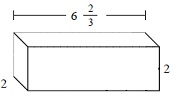### Explanation

Step 1:

Solid of cubes with unit fraction edge lengths.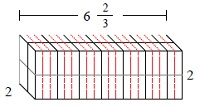Step 2:

Volume V = l w h = $6 \frac{2}{3}$ × 2 × 2

= $26 \frac{2}{3}$ cu cm